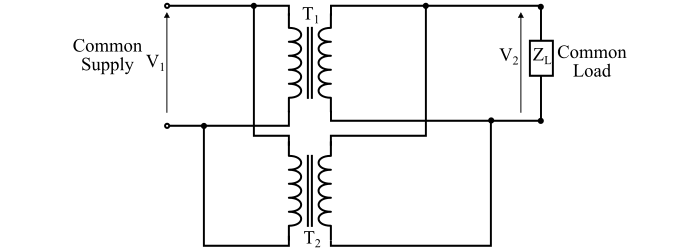# Parallel Operation of Transformers – Reasons and Conditions

When the primary windings of two or more transformers are connected to a common voltage supply and their secondary windings are connected to a common load. Then, the transformers are said to be connected in parallel, i.e., parallel operation of the transformers. The figure shows two transformers T1 and T2 connected in parallel.## Reasons for Parallel Operation of Transformers

The primary reasons for operating transformers in parallel are as follows −

• For large loads, it may be impractical or uneconomical to have a single large transformer so that many small transformers are paralleled to meet the load demand.

• By operating the transformers of standard size in parallel at the substations, the spare capacity of the substation can be reduced.

• There is always a scope of future expansion of a substation to supply a load of capacity greater than that of the transformers already installed. Hence, in future by connecting a new transformer in parallel with existing transformers, the total capacity of the substation can be increased.

• If a transformer is damaged in a system of transformers connected in parallel and is removed for repair and maintenance, then there is no interruption of power supply for essential services.

## Conditions for Parallel Operation of Single-Phase Transformers

### Necessary Conditions

For satisfactory parallel operation of the transformers, two main conditions are necessary −

• The parallel connected transformers must be of the same polarities. Otherwise, huge circulating currents flow in the windings.

• The voltage ratios must be equal of the parallel connected transformers. If the voltage ratios are not same, then the secondaries will not show the same voltage even if the primaries are connected to the same voltage supply. As a result of this, a current circulates in the secondaries and hence there will also be circulating currents on the primary side. Due to this, a considerable amount of current is drawn by the transformers even without the load.

### Desirable Conditions

For efficient parallel operation of the transformers, following conditions are desirable −

• The internal impedances of the parallel connected transformers should be equal.

• The ratios of the winding reactances to the resistances should be equal for all the parallel connected transformers. This ensures that the transformers operate on the same power factor, hence, sharing the active and reactive powers according to their ratings.

## Conditions for Parallel Operation of Three-Phase Transformers

The conditions for satisfactory parallel operation of three-phase transformers are as follows −

• The parallel connected transformers should have same polarities.

• The parallel connected transformers must have identical primary and secondary voltage ratings.

• The winding reactances to the resistances ratios in the parallel connected transformers should be the same.

• The phase sequence of all the parallel connected transformers must be the same.

• The phase shift between the primary and secondary voltages must be the same for all the parallel connected transformers.

• All the parallel connected transformers should be in the same vector group.